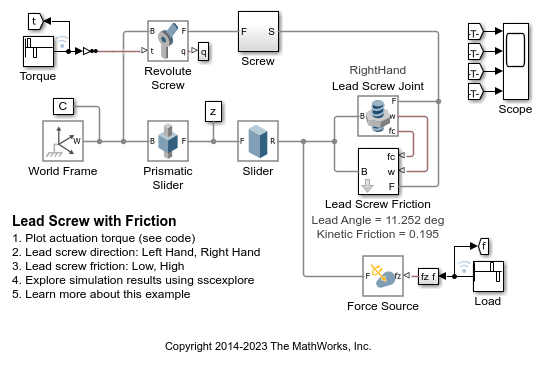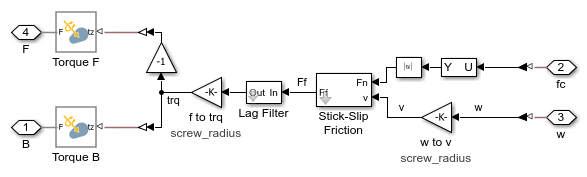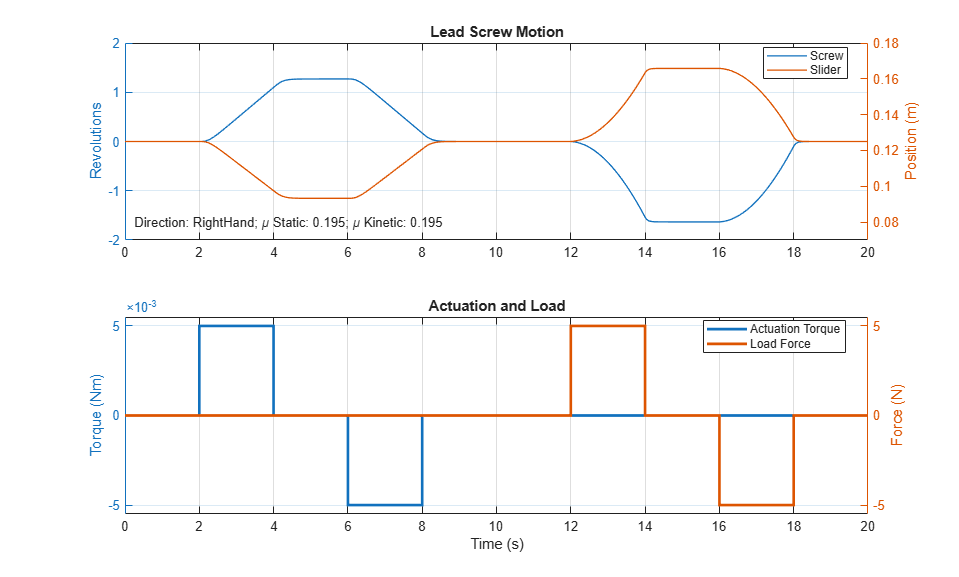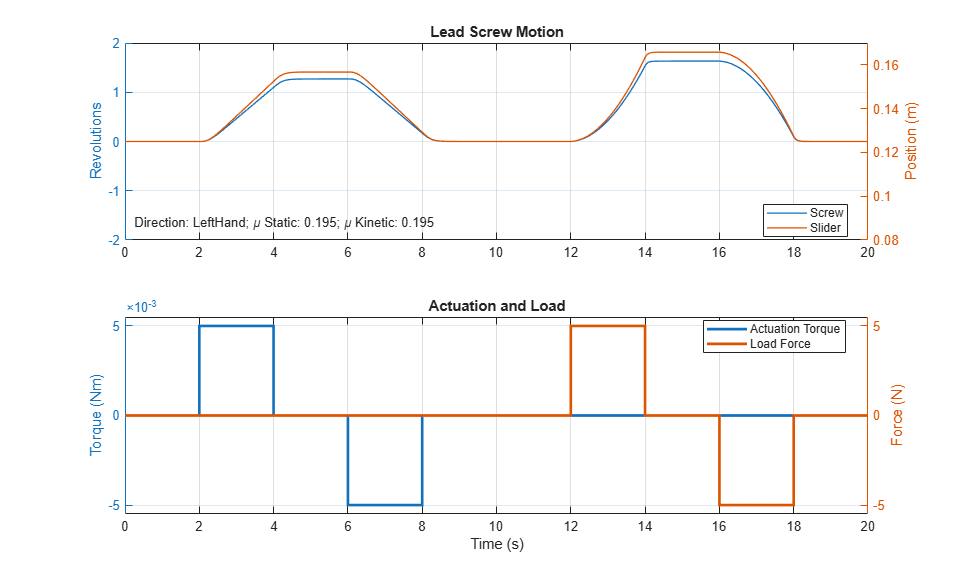This example models a lead screw with friction. The constraint force in the lead screw is measured and used to calculate the friction torque within the lead screw. A continuous stick-slip friction model is used to determine the coefficient of friction based on the relative rotational speed of the two parts connected by the lead screw.

### ModelThis subsystem calculates and applies the friction torque to the two parts connected by the lead screw joint. Open Subsystem### Simulation Results from Simscape Logging

The plot below shows the actuation torque of the lead screw. In this test, the coefficient of friction is low enough that the load force can backdrive the lead screw.The Lead Screw Joint can be configured such that positive rotation leads to positive translation.Increasing the coefficient of friction higher than the tangent of the lead angle will make the lead screw non-backdriveable. Applying an axial load force will not be sufficient for the screw to move.